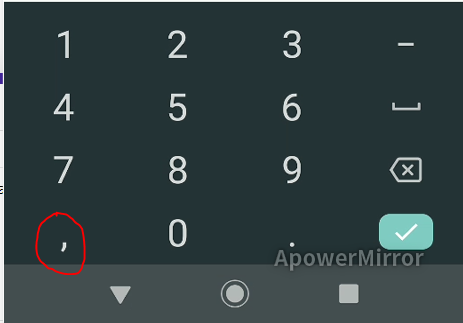# Don't let user type comma instead of period

My calculation only accepts the point as a floating point. How do I show the user a message that the calculation only accepts a point instead of a comma. Example: Weight = 72.3 instead of 72,3. Height = 1.57 instead of 1,57. I left an example in the textbox hint but the user still forgets it.

User will type the numbers in text box

So On text changed block
Use if contains , then either alert user or replace the comma with .

I did it that way, but when I try to type the comma, it doesn’t accept it. It does the operation but converts the value that was placed to an integer. Example: if you try to type 1.57 it won’t let you type the comma. When I put the point it accepts but when doing the calculation the result comes out correct but the value 1.57 changes to 2949 (integer) and the message does not appear. Can you help me?

you mean problem with decimal value not appearing properly??

I’ll run the application and show you what’s going on

When trying to type the number 1,57 (one comma fifty-seven) the comma on the numeric keyboard is not accepted. When I type with a dot it accepts, does the calculation but converts the decimal number into an integer.

interchange the order and try

I mean let the set Height text value come after calculating the IMC text value else your calculation will go less

I won’t need to pass message or rule. When opening the numerical keyboard, there is no way to type the comma only period.Another question: I know that Niotron has a forum but most of my questions I get on the Kodular forum. Why does it happen? They don’t know how to solve it?